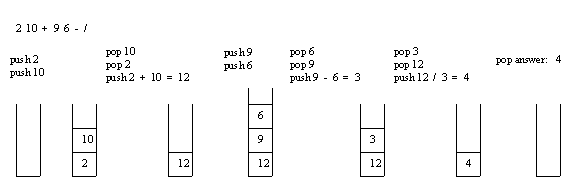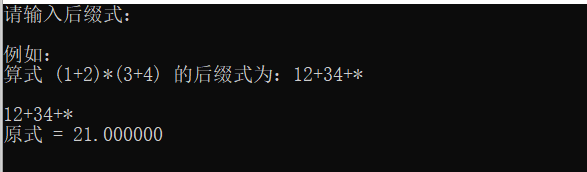• 2021-05-20 12:37:48

::iterator b = v.begin(); b < v.end(); b++)

{

strcat(infix, (*b).c_str());

}

return infix;

}

// 计算后缀表达式的值，仅支持加减乘除运算、操作数为非负整数的表达式。

int postfix_eval(const char * postfix)

{

const size_t N = strlen(postfix);

if (N == 0)

{

return 0;

}

STACKoperand(N); // 堆栈存放的是操作数

for (size_t i = 0 ; i < N; i++)

{

switch (postfix[i])

{

int op1, op2;

case ''+'':

op1 = operand.pop();

op2 = operand.pop();

operand.push(op1 + op2);

break;

case ''-'':

op1 = operand.pop();

op2 = operand.pop();

operand.push(op1 - op2);

break;

case ''*'':

op1 = operand.pop();

op2 = operand.pop();

operand.push(op1 * op2);

break;

case ''/'':

op1 = operand.pop();

op2 = operand.pop();

operand.push(op1 / op2);

break;

default:

if (isdigit(postfix[i])) // 执行类似atoi()的功能

{

operand.push(0);

while (isdigit(postfix[i]))

{

operand.push(10 * operand.pop() + postfix[i++] - ''0'');

}

i--;

}

}

std::cout << operand << std::endl; // 输出堆栈的内容

}

return operand.pop();

}

// 本程序演示了如何后缀表达式和中缀表达式的相互转换，并利用堆栈计算后缀表达式。

// 转换方向：org_infix --> postfix --> infix

int main(int argc, const char *argv[])

{

// const char *org_infix = "(5*(((9+8)*(4*6))+7))"; // section 4.3

const char *org_infix = "(5*((9*8)+(7*(4+6))))"; // exercise 4.12

std::cout << "原始中缀表达式：" << org_infix << std::endl;

char *const postfix = new char[strlen(org_infix) + 1];

infix2postfix(org_infix, postfix);

std::cout << "后缀表达式：" << postfix << std::endl;

char *const infix = new char[strlen(postfix) + 1];

postfix2infix(postfix, infix);

std::cout << "中缀表达式：" << infix << std::endl;

std::cout << "计算结果是：" << postfix_eval(postfix) << std::endl;

std::cout << "计算结果是：" << postfix_eval("5 9*8 7 4 6+*2 1 3 * + * + *") << std::endl; // exercise 4.13

delete []infix;

delete []postfix;

return 0;

}

更多相关内容
• 后缀表达式求值c语言算法Here you will get algorithm and program for evolution of postfix expression in C. 在这里，您将获得用于C语言后缀表达式演变的算法和程序。 In postfix or reverse polish notation...后缀表达式求值c语言算法

Here you will get algorithm and program for evolution of postfix expression in C.

在这里，您将获得用于C语言中后缀表达式演变的算法和程序。

In postfix or reverse polish notation, every operator follows all of its operands.

使用后缀或反向抛光表示法，每个运算符都将遵循其所有操作数。

For example: 5 3 2 * +

例如： 5 3 2 * +

Also Read: Infix to Postfix Conversion in C [Program and Algorithm]

## 后缀表达评估算法 (Algorithm for Evaluation of Postfix Expression)

Create an empty stack and start scanning the postfix expression from left to right.

创建一个空堆栈，然后开始从左到右扫描后缀表达式。

• If the element is an operand, push it into the stack.

如果元素是操作数，则将其压入堆栈。

• If the element is an operator O, pop twice and get A and B respectively. Calculate BOA and push it back to the stack.

如果元素是运算符O ，则弹出两次并分别获得A和B。 计算B O A并将其推回堆栈。

• When the expression is ended, the value in the stack is the final answer.

表达式结束时，堆栈中的值是 最终答案。

Evaluation of a postfix expression using a stack is explained in below example:

下面的示例说明了使用堆栈对后缀表达式的求值：## C语言中后缀表达的评估程序 (Program for Evaluation of Postfix Expression in C)

//Assumption -- primary operators '-,+,*,/,%' operand -- a single digit

#include<stdio.h>

#define MAX 20

typedef struct stack
{
int data[MAX];
int top;
}stack;

void init(stack *);
int empty(stack *);
int full(stack *);
int pop(stack *);
void push(stack *,int);
int evaluate(char x,int op1,int op2);

int main()
{
stack s;
char x;
int op1,op2,val;
init(&s);
printf("Enter the expression(eg: 59+3*)\nSingle digit operand and operators only:");

while((x=getchar())!='\n')
{
if(isdigit(x))
push(&s,x-48);		//x-48 for removing the effect of ASCII
else
{
op2=pop(&s);
op1=pop(&s);
val=evaluate(x,op1,op2);
push(&s,val);
}
}

val=pop(&s);
printf("\nValue of expression=%d",val);

return 0;
}

int evaluate(char x,int op1,int op2)
{
if(x=='+')
return(op1+op2);
if(x=='-')
return(op1-op2);
if(x=='*')
return(op1*op2);
if(x=='/')
return(op1/op2);
if(x=='%')
return(op1%op2);
}

void init(stack *s)
{
s->top=-1;
}

int empty(stack *s)
{
if(s->top==-1)
return(1);

return(0);
}

int full(stack *s)
{
if(s->top==MAX-1)
return(1);

return(0);
}

void push(stack *s,int x)
{
s->top=s->top+1;
s->data[s->top]=x;
}

int pop(stack *s)
{
int x;
x=s->data[s->top];
s->top=s->top-1;

return(x);
}

Output

输出量

Enter the expression(eg: 59+3*) Single digit operand and operators only:74+5-

输入表达式(例如：59 + 3 *) 仅一位数字的操作数和运算符：74 + 5-

Value of expression=6

表达式的值= 6

后缀表达式求值c语言算法

展开全文算法 堆栈 stack
• 实现数据结构中后缀表达式值c语言完整可运行代码。
• c语言实现中缀表达式转后缀表达式并求得计算结果，用顺序栈结构。 当输入者输入错误信息的时候需要报错，并说明错误的种类。
• 本文实例为大家分享了C语言实现中缀表达式转后缀表达式的具体代码，供大家参考，具体内容如下 中缀表达式转换为后缀表达式（思路） 1.创建栈 2.从左向右顺序获取中缀表达式 a.数字直接输出 b.运算符 情况一：遇到左...
• C语言 实现后缀表达式求值（理解不同方式）

# 写在前面

在数据结构中，栈是非常重要的储存结构，比如栈应用于求表达式，将输入的等式进行转换，如前缀表达式、后缀表达式等然后进行求值并返回结果。

但是本文并为使用栈实现后缀表达式求值，而是通过一般函数方法，需要先计算一下，将原式转换成后缀表达式后再输入求值哦，试试看。

# 代码实现

#include <stdio.h>
#include <stdlib.h>

void evalPf(char*);
double cal(double, char, double);

int main(){
char input;
printf("请输入后缀式：\n\n");
printf("例如：\n算式 (1+2)*(3+4) 的后缀式为：12+34+*  \n\n");
scanf("%s", input);
evalPf(input);
return 0;
}

void evalPf(char* postfix){
double stack = {0.0};
char temp;
char token;
int top = 0, i = 0;
temp = '\0';
while(1){
token = postfix[i];
switch(token){
case '\0':
printf("原式 = %f\n", stack[top]);
return;
case '+':
case '-':
case '*':
case '/':
stack[top-1] =cal(stack[top], token, stack[top-1]);
top--;
break;
default:
if(top < sizeof(stack) / sizeof(float)){
temp = postfix[i];
top++;
stack[top] = atof(temp);
}
break;
}
i++;
}
}

double cal(double p1, char op, double p2){
switch(op){
case '+':
return p1 + p2;
case '-':
return p1 - p2;
case '*':
return p1 * p2;
case '/':
return p1 / p2;
}
}

# 运行结果展开全文数据结构 算法
• ​ 上次已经完成了由中缀表达式转后缀表达式的算法，而后缀表达式的优点就是可以从左至右直接读取，没有算数优先级的考量，所以直接进行运算即可。 ​ 该算法需要使用一个栈用来保存操作数，在读取到数字的时候，将...

## 算法思路

​ 上次已经完成了由中缀表达式转后缀表达式的算法，而后缀表达式的优点就是可以从左至右直接读取，没有算数优先级的考量，所以直接进行运算即可。

​ 该算法需要使用一个栈用来保存操作数，在读取到数字的时候，将数字压入栈中；如果读取到操作符，就弹出栈顶的两个元素，分别为op2和op1（先弹出来的元素为op2），将op1和op2与目前读取到的操作符进行计算，并将结果压回栈中。反复运行直到后缀表达式全部读取完成，此时栈中的结果即后缀表达式的结果。

## 具体过程

例后缀表达式12+3-45*2/+，定义一个栈num来保存数字，顺序读取后缀表达式。

1. 读取到数字1，将其压入栈中，此时num = ['1']
2. 读取到数字2，将其压入栈中，此时num = ['1','2']
3. 读取到操作符+，将栈顶的两个元素弹出，op2=2op1=1，将op1+op2的结果压入栈中，此时num = ['3']
4. 读取到数字3，将其压入栈中，此时num = ['3','3']
5. 读取到操作符-，将栈顶的两个元素弹出，op2=3op1=3，将op1-op2的结果压入栈中，此时num = ['0']
6. 读取到数字4，将其压入栈中，此时num = ['0','4']
7. 读取到数字5，将其压入栈中，此时num = ['0','4','5']
8. 读取到操作符*，将栈顶的两个元素弹出，op2=5op1=4，将op1*op2的结果压入栈中，此时num = ['0','20']
9. 读取到数字2，将其压入栈中，此时num = ['0','20','2']
10. 读取到操作符/，将栈顶的两个元素弹出，op2=2op1=20，将op1/op2的结果压入栈中，此时num = ['0','10']
11. 读取到操作符+，将栈顶的两个元素弹出，op2=10op1=0，将op1+op2的结果压入栈中，此时num = ['10']
12. 后缀表达式读取完毕，此时栈num的内容即后缀表达式的结果。

## 代码实现

// 后缀表达式求值
void postfixEval()
{
char postfixList = {0};    // 使用char类型数组保存后缀表达式
printf("请输入将要求值的后缀表达式:\n");
scanf("%s", postfixList);
getchar();
int length;
length = (int) strlen(postfixList);
LiStack num;    // 定义栈num保存数字，并定义
num = initStack();

for (int i = 0; i < length; i++)
{
if (isdigit(postfixList[i]))
{
int nums = asciiToint(postfixList[i]);  // 因为在char类型中保存数字是ascii码类型，所以需要将其转义，具体函数在下方
push(num, nums);    // 将数字压入栈中
}
else
{
int second = pop(num);
int first = pop(num);
push(num, domatch(first, postfixList[i], second));  // 调用domatch（定义在下方），将对应结果再次压入栈中
}
}
printf("该表达式的值为%d\n", pop(num));
}

// 将ascii码转化为整形

int asciiToint(char ascii)
{
// 因为在ascii码中，48表示0，49表示1……将ascii-'0'，就是将对应数字的ascii码减去48，结果就是十进制中的数字
int i = ascii - '0';
return i;
}

// 算数求值
int domatch(int op1, char op, int op2)  // 将char类型的运算符进行相应的运算
{
int first = (int)op1, second = (int)op2;
if (op == '*')
{
return first * second;
}
else if(op == '/')
{
return first / second;
}
else if (op == '+')
{
return first + second;
}
else
{
return first - second;
}
}


展开全文算法 数据结构
• 本文实例为大家分享了C++利用链栈实现表达式求值的具体代码，供大家参考，具体内容如下 #include typedef int Status; typedef char Cstack; #define OK 1 #define ERROR 0 typedef struct StackNode { Cstack ...
• 我们平时数学上用的算术表达式（中缀表达式）转换为后缀表达式后，它主要是字符串形式（如果不会，建议先学习中转后的操作）。 要实现对其的求值，有如下几个步骤： （对整个字符串进行遍历） 将字符串型的数字转换...
• 注：最主要需要注意的是代码中那个标注的getchar（）函数的使用 #include<stdio.h> #include<stdlib.h> #include<math.h> #define False 0 #define True 1 typedef struct ...ma数据结构 stack
• 要求设计类（或类模板）来描述算术表达式及其操作，包含必要的构造函数和析构函数，以及其他能够完成如下功能的成员函数：输入算术表达式（中缀表示）中缀表达式转换为后缀表达式输出表达式对后缀表达式求值
• 更改了一下中缀表达式转后缀表达式以及后缀表达式求值的算法，加入了对小数、负数的支持，注释完整，也可随时联系我。
• 2、后缀表达式求值 合并 */ #include<stdlib.h> #include<stdio.h> #define Maxsize 10 typedef struct node{ char data[Maxsize]; int top; }Stack; bool Isempty(Stack &s){ if(s.top == -1...数据结构 算法
• 后缀表达式什么是后缀表达式后缀表达式计算功能快捷键合理的创建标题，有助于目录的生成如何改变文本的样式插入链接与图片如何插入一段漂亮的代码片生成一个适合你的列表创建一个表格设定内容居中、居左、居右...
• } //表达式运算符优先级低则执行出栈操作，弹出的栈顶元素成为后缀表达式的一部分 //当遇到“）”，则执行出栈操作直到“（”，但括号不计入后缀表达式中 while (!IsEmpty(Stack) && JudgeLevel(0, a[i]) (1, Stack...stack 数据结构
• ## 后缀表达式的求值（c语言）

万次阅读 多人点赞 2018-06-29 19:50:59
后缀表达式的处理过程如下：扫描后缀表达式，凡遇操作数则将之压进堆栈，遇运算符则从堆栈中弹出两个操作数进行该运算，将运算结果压栈，然后继续扫描，直到后缀表达式被扫描完毕为止，此时栈底元素即为该后缀表...
• 【步骤】表达式–>后缀表达式–>求值 表达式转后缀表达式后缀表达式求值： 表达式转后缀表达式 步骤 Stack OPND; //存储后缀【表达式】的栈 Stack OPTR; //存储【符号】的栈 OPTR.push('...
• 表达式求值c语言版，能后缀，并根据后缀求表达式。仅供参考与交流
• 一、先将中缀表达式转为后缀表达式 规则： 遇到数字：直接输出 遇到左括号：直接入栈 遇到右括号：输出栈顶元素，直至遇到左括号或者栈空；右括号不入栈 遇到运算符：分两种情况：i）当前运算符优先级大于栈顶...中缀表达式
• C语言计算后缀表达式，从控制台输入，以空格为分割输入，支持多位数和负数运算，支持加、减、乘、除、阶乘操作，支持表达式错误检测异常。 input： 3 1 -2 * 1 10 - 6 + 3 1 - ！ Output： -2 -3 2 #include<...开发语言
• C语言中，将中缀表达式转成后缀表达式，并计算它的结果，具体的思路如下： 1、定义两个栈，numStack用于存放运算对象，最后存放运算结果的，fuStack用于存放运算符号的 2、从左到右遍历字符串 3、如果当前的字符是...
• 首先需搞懂什么是后缀表达式，这个可以找一些视频会更容易理解，然后下面是具体的代码实现： 操作数在0-9之间且输入的字符串无空格，如“897*+63/+”，代码如下： #include<bits/stdc++.h> using namespace...数据结构 c++ 算法
• 后缀表达式求值 【问题描述】 读入一个后缀表达式（字符串），只含有0-9组成的运算数及 加（+）、减（−）、乘（∗）、除（/，整除）四种运算符，以及空格。 每个运算数之间用一个空格隔开，不需要判断给你的表达式...c++ 算法
• 对逆波兰式（即后缀表达式求值。其中每个操作数的取值范围为0~9，均为个位数。运算符为加减乘除四种（+、-、*、/）。如（4+5）2-3的逆波兰式为45+23-。 输入说明： 一个字符串表示的逆波兰式，如：45+2*3-，表达式...链表
• 后缀表达式求值 首先需要将输入的中缀表达式转换成后缀表达式后缀表达式中，操作符在后 例如：1+2*(5-3)+4 后缀表达式：1253-*+4+ 1、中缀表达式转换成后缀表达式方法： 首先，需要定义一个操作符栈并且知道各...数据结构
• 此程序先将输入的中缀表达式转化为后缀表达式，然后计算后缀表达式，此为栈的应用。
• 假定运算符集合为{ +、-、*、/、（、）}，利用栈将输入的中缀表达式转换成等价的后缀表达式，并输出。 输入格式: 输入一个字符串表示的中缀表达式（以“#”结束），其中每个操作数用一个小写字母表示。 输出格式: 将......